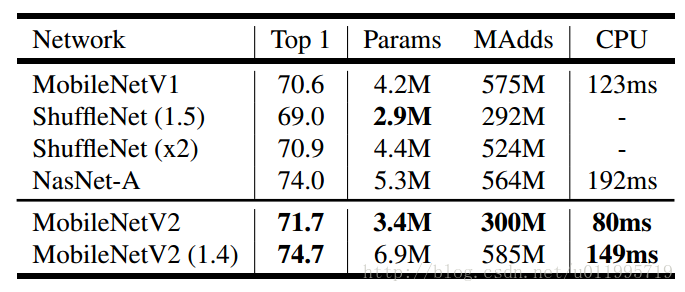# 我的模型有多快？——深度学习网络模型的运算复杂度、空间占用和内存访问情况计算

• 空间占用——单个模型的参数文件要占用多大空间
• 内存占用——运行在手机或平板上时需要占用多大的 RAM
• 运行速度——尤其考虑实时的视频和大图像处理情形
• 耗电情况——我可不想要暖手宝

## 正文

（网上随手找的图：https://blog.csdn.net/u011995719/article/details/79135818（注：可参考

### 1.计算消耗

y = w*x + w*x + w*x + ... + w[n-1]*x[n-1]

wx 都是向量，y 是标量。上式是全连接层或卷积层的典型运算。一次乘-加运算即一次乘法+一次加法运算，所以上式的 MACCs 是n

#### 1.1 全连接层

y = matmul(x, W) + b

#### 1.2 激活函数

FC 完了接下来通常有个激活函数，ReLU 或者 Sigmoid。激活函数的计算没有点积，所以只用 FLOPS 衡量。

y = max(x, 0)

y = 1/(1+exp(-x))

#### 1.3 卷积层

K×K×Cin×Hout×Wout×Cout K × K × C i n × H o u t × W o u t × C o u t <script id="MathJax-Element-31" type="math/tex">K \times K \times Cin \times Hout \times Wout \times Cout</script>

• 输出的 feature map 里每个通道上有 Hout×Wout H o u t × W o u t <script id="MathJax-Element-32" type="math/tex">Hout \times Wout</script> 个元素，
• 权重以 K×K K × K <script id="MathJax-Element-33" type="math/tex">K \times K</script> 大小的窗口，在所有的 Cin C i n <script id="MathJax-Element-34" type="math/tex">Cin</script> 个通道上做点积，
• 共有 Cout C o u t <script id="MathJax-Element-35" type="math/tex">Cout</script> 个卷积核，上述操作重复了 Cout C o u t <script id="MathJax-Element-36" type="math/tex">Cout</script> 次

3×3 3 × 3 <script id="MathJax-Element-38" type="math/tex">3 \times 3</script> 卷积，128 个 filer，输入的 feature map 是 112×112×64 112 × 112 × 64 <script id="MathJax-Element-39" type="math/tex">112 \times 112 \times 64</script> ，stride=1padding=same，MACCs 有：

3×3×64×112×112×128=924,844,032 3 × 3 × 64 × 112 × 112 × 128 = 924 , 844 , 032 <script id="MathJax-Element-40" type="math/tex">3 \times 3 \times 64 \times 112 \times 112 \times 128 = 924, 844, 032</script>

#### 1.4 Batch Normalization

z = gamma * (y - mean) / sqrt(variance + epsilon) + beta

（ BN 层的计算结合到 Conv 层中去，BN 层的 FLOPS 消失了，Conv 层需要乘一个常系数）

#### 1.5 其他层

RNN 这里不做讨论。简单来说，以 LSTM 为例，计算主要是两个大的矩阵乘法，sigmoid，tanh 和一些元素级的操作。可以看成两个全连接层的运算，所以 MACCs 主要取决于输入、输出和隐状态向量的尺寸。点积运算还是占了大头。

### 2. 内存占用

• 从主内存中读取输入向量 / feature map
• 从主内存中读取权重并计算点积
• 将输出向量或 feature map 写回主内存

#### 2.1 权重的内存占用

3 x 3 、48个卷积核，在64x64 、32个通道的输入上计算，共有 3 x 3 x 32 x 48 + 48 = 13, 872 个权重。

#### 2.2 feature maps 和中间结果

CS231n 的 Lesson 9 专门花了很多篇幅讲 feature map 的计算，可以参考。

input = Hin x Win x Cin x K x K x Cout

output = Hout x Wout x Cout

weights = K x K x Cin x Cout + Cout ，按上例：

input = 224 x 224 x 3 x 3 x 3 x 32 = 43,352,064
output = 112 x 112 x 32 = 401,408
weights = 3 x 3 x 3 x 32 + 32 = 896
total = 43,754,368

input = 28 x 28 x 256 x 3 x 3 x 512 = 924,844,032
output = 28 x 28 x 512 = 401,408
weights = 3 x 3 x 256 x 512 + 512 = 1,180,160
total = 926,425,600

### 3. MobileNet V2 vs. V1

V2 with multiplier=1.4 的速度略慢于 V1，但精度高出不少；V2 with multiplier=1.0 速度比 V1 快很多。可以根据需要进行取舍。官方页面上也给了很多实验参考。

VGG16 经常被当作图像方面的特征提取器，结构很简单，层数也不多，看起来好像计算比较多、内存访问会少一些，真的是这样吗？对比 MobileNet（输入按移动设备16:9的规格，是126x224，可以算出以下结果：

VGG16 params: 15M
VGG16 MACCs : 8380M
VGG16 MAes  : 8402M

### 进一步阅读

03-146721
01-181万+04-282310
09-12882
07-154475
10-314317
03-24135
05-06832
08-102万+